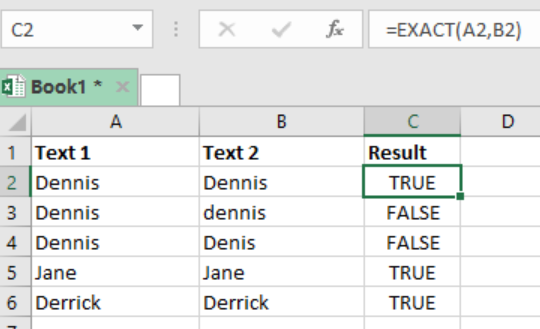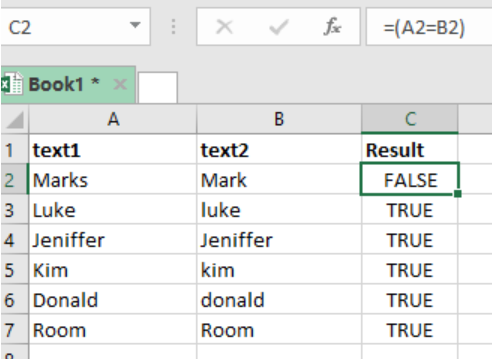Get instant live expert help with Excel or Google Sheets“My Excelchat expert helped me in less than 20 minutes, saving me what would have been 5 hours of work!”

#### Post your problem and you’ll get Expert help in seconds.

Your message must be at least 40 characters
Our professional Expert are available now. Your privacy is guaranteed.

# Excel EXACT Function

As we work with Excel spreadsheets, we might want to compare text strings. The Excel EXACT function can help compare two text strings, putting into account upper and lower case characters, given that the function is case-sensitive. This post provides a clear guide on how to use the Excel EXACT function when working with Excel spreadsheet.Figure 1: Using EXACT function to match text strings

## Syntax of the formula

`=EXACT(text1, text2)`

Where;

Text1- refers to the first text string to compare

Text2- refers to the second text string to compare

## Explanation of the formula

The EXACT function will return TRUE if the two text strings being compared are same, and FALSE if they are not. Remember that this function is case-sensitive, and thus if there is variation in the cases used, then it will return FALSE even if the text strings are same.

In our example above, we have used the following formula in cell C2;

`=EXACT(A2,B2)`

We then copy down the formula to get results of the other cells.

If you want to ignore the case sensitive nature of the EXACT function, then you can use the equal sign, which is not case-sensitive, i.e. (text1=text2)

## Example: Use of equal signFigure 2: Ignoring lower and upper cases.

In this example, we have use the formula below in cell C2;

`=(A2=B2)`

You will then notice that unlike in our first example, this one is not case-sensitive and r=will return TRUE for all text strings that mean the same, ignoring upper or lower cases.

Most of the time, the problem you will need to solve will be more complex than a simple application of a formula or function. If you want to save hours of research and frustration, try our live Excelchatservice! Our Excel Experts are available 24/7 to answer any Excel question you may have. We guarantee a connection within 30 seconds and a customized solution within 20 minutes.

### Did this post not answer your question? Get a solution from connecting with the expert.Another blog reader asked this question today on Excelchat:
Solution examplesUse the Vlookup Function to complete the "employee" column of table 2. Use "job Id" from table 2 as your lookup_value(s) and table 1 as your reference.
Solved by C. H. in 16 minsIf a cell in another sheet is populated I need a vlookup done. If the cell is not populated I need the cell to return blank.
Solved by T. D. in 60 minsI am trying to make a chart that turns a week range red if nothing is entered in the range. If something is entered then I would like it to turn green. Please Help
Solved by E. U. in 43 minsI need a check box to show/hide an answer of an if function
Solved by Z. U. in 23 minsI need a formula to compare the data in two columns and then export the mismatched data in the 3rd column
Solved by S. Q. in 20 mins## Subscribe to Excelchat.coAnother blog reader asked this question today on Excelchat: